# The Universe of Discourse

Fri, 16 Feb 2007

Yahtzee probability
In the game of Yahtzee, the players roll five dice and try to generate various combinations, such as five of a kind, or full house (a simultaneous pair and a three of a kind.) A fun problem is to calculate the probabilities of getting these patterns. In Yahtzee, players get to re-roll any or all of the dice, twice, so the probabilities depend in part on the re-rolling strategy you choose. But the first step in computing the probabilities is to calculate the chance of getting each pattern in a single roll of all five dice.

A related problem is to calculate the probability of certain poker hands. Early in the history of poker, rules varied about whether a straight beat a flush; players weren't sure which was more common. Eventually it was established that straights were more common than flushes. This problem is complicated by the fact that the deck contains a finite number of each card. With cards, drawing a 6 reduces the likelihood of drawing another 6; this is not true when you roll a 6 at dice.

With three dice, it's quite easy to calculate the likelihood of rolling various patterns:

 Pattern Probability A A A 6 / 216 A A B 90 / 216 A B C 120 / 216

A high school student would have no trouble with this. For pattern AAA, there are clearly only six possibilities. For pattern AAB, there are 6 choices for what A represents, times 5 choices for what B represents, times 3 choices for which die is B; this makes 90. For pattern ABC, there are 6 choices for what A represents times 5 choices for what B represents times 4 choices for what C represents; this makes 120. Then you check by adding up 6+90+120 to make sure you get 63 = 216.

It is perhaps a bit surprising that the majority of rolls of three dice have all three dice different. Then again, maybe not. In elementary school I was able to amaze some of my classmates by demonstrating that I could flip three coins and get a two-and-one pattern most of the time. Anyway, it should be clear that as the number of dice increases, the chance of them all showing all different numbers decreases, until it hits 0 for more than 6 dice.

The three-die case is unusually simple. Let's try four dice:

 Pattern Probability A A A A 6 / 1296 A A A B 120 / 1296 A A B B 90 / 1296 A A B C 720 / 1296 A B C D 360 / 1296

There are obviously 6 ways to throw the pattern AAAA. For pattern AAAB there are 6 choices for A × 5 choices for B × 4 choices for which die is the B = 120. So far this is no different from the three-die case. But AABB has an added complication, so let's analyze AAAA and AAAB a little more carefully.

First, we count the number of ways of assigning numbers of pips on the dice to symbols A, B, and so on. Then we count the number of ways of assigning the symbols to actual dice. The total is the product of these. For AAAA there are 6 ways of assigning some number of pips to A, and then one way of assigning A's to all four dice. For AAAB there are 6×5 ways of assigning pips to symbols A and B, and then four ways of assigning A's and B's to the dice, namely AAAB, AABA, ABAA, and BAAA. With that in mind, let's look at AABB and AABC.

For AABB, There are 6 choices for A and 5 for B, as before. And there are !!4\choose2!! = 6 choices for which dice are A and which are B. This would give 6·5·6 = 180 total. But of the 6 assignments of A's and B's to the dice, half are redundant. Assignments AABB and BBAA, for example, are completely equivalent. Taking A=2 B=4 with pattern AABB yields the same die roll as A=4 B=2 with pattern BBAA. So we have double-counted everything, and the actual total is only 90, not 180.

Similarly, for AABC, we get 6 choices for A × 5 choices for B × 4 choices for C = 120. And then there seem to be 12 ways of assigning dice to symbols:

 AABC AACB ABAC ACAB ABCA ACBA BAAC CAAB BACA CABA BCAA CBAA

But no, actually there are only 6, because B and C are entirely equivalent, and so the patterns in the left column cover all the situations covered by the ones in the right column. The total is not 120×12 but only 120×6 = 720.

Then similarly for ABCD we have 6×5×4×3 = 360 ways of assigning pips to the symbols, and 24 ways of assigning the symbols to the dice, but all 24 ways are equivalent, so it's really only 1 way of assigning the symbols to the dice, and the total is 360.

The check step asks if 6 + 120 + 90 + 720 + 360 = 64 = 1296, which it does, so that is all right.

Before tackling five dice, let's try to generalize. Suppose the we have N dice and the pattern has kN distinct symbols which occur (respectively) p1, p2, ... pk times each.

There are !!{6\choose k}k!!! ways to assign the pips to the symbols. (Note for non-mathematicians: when k > 6, !!{6\choose k}!! is zero.)

Then there are !!N\choose p_1 p_2 \ldots p_k!! ways to assign the symbols to the dice, where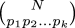denotes the so-called multinomial coefficient, equal to !!{N!\over p_1!p_2!\ldots p_k!}!!.

But some of those pi might be equal, as with AABB, where p1 = p2 = 2, or with AABC, where p2 = p3 = 1. In such cases case some of theassignments are redundant.

So rather than dealing with the pi directly, it's convenient to aggregate them into groups of equal numbers. Let's say that ni counts the number of p's that are equal to i. Then instead of having pi = (3, 1, 1, 1, 1) for AAABCDE, we have ni = (4, 0, 1) because there are 4 symbols that appear once, none that appear twice, and one ("A") that appears three times.

We can re-express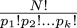in terms of the ni:

$$N!\over {1!}^{n_1}{2!}^{n_2}\ldots{k}!^{n_k}$$

And the reduced contribution from equivalent patterns is easy to express too; we need to divide by !!\prod {n_i}!!!. So we can write the total as:

$${6\choose k}k! {N!\over \prod {i!}^{n_i}{n_i}!} \qquad \text{where k = \sum n_i}$$

Note that k, the number of distinct symbols, is merely the sum of the ni.

To get the probability, we just divide by 6N. Let's see how that pans out for the Yahtzee example, which is the N=5 case:

 Pattern ni Probability 1 2 3 4 5 A A A A A 1 6 / 7776 A A A A B 1 1 150 / 7776 A A A B B 1 1 300 / 7776 A A A B C 2 1 1200 / 7776 A A B B C 1 2 1800 / 7776 A A B C D 3 1 3600 / 7776 A B C D E 5 720 / 7776

6 + 150 + 300 + 1,200 + 1,800 + 3,600 + 720 = 7,776, so this checks out. The table is actually not quite right for Yahtzee, which also recognizes "large straight" (12345 or 23456) and "small straight" (1234X, 2345X, or 3456X.) I will continue to disregard this.

The most common Yahtzee throw is one pair, by a large margin. (Any Yahtzee player could have told you that.) And here's a curiosity: a full house (AAABB), which scores 25 points, occurs twice as often as four of a kind (AAAAB), which scores at most 29 points and usually less.

The key item in the formula is the factor of !!{N!\over \prod {i!}^{n_i}{n_i}!}!! on the right. This was on my mind because of the article I wrote a couple of days ago about counting permutations by cycle class. The key formula in that article was:

 BuyConcrete Mathematicsfrom Bookshop.org(with kickback)(without kickback)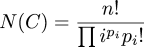which has a very similar key item. The major difference is that instead of i!ni we have ipi. The common term arises because both formulas are intimately concerned with the partition structure of the things being counted. I should really go back and reread the stuff in Concrete Mathematics about the Stirling numbers of the first kind, which count the number of partitions of various sizes, but maybe that's a project for next week.

Anyway, I digress. We can generalize the formula above to work for S-sided dice; this is a simple matter of replacing the 6 with an S. We don't even need to recalculate the ni. And since the key factor of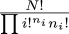does not involve S, we can easily precalculate it for some pattern and then plug it into the rest of the formula to get the likelihood of rolling that pattern with different kinds of dice. For example, consider the two-pairs pattern AABBC. This pattern has n1 = 1, n2 = 2, so the key factor comes out to be 15. Plugging this into the rest of the formula, we see that the probability of rolling AABBC with five S-sided dice is !!90 {S \choose 3} S^{-5}!!. Here is a tabulation:

 # ofsides Chance ofrolling AABBC 3 37.03704 % 4 35.15625 5 28.80000 6 23.14815 7 18.74219 8 15.38086 9 12.80293 10 10.80000 20 3.20625 50 0.56448 100 0.14553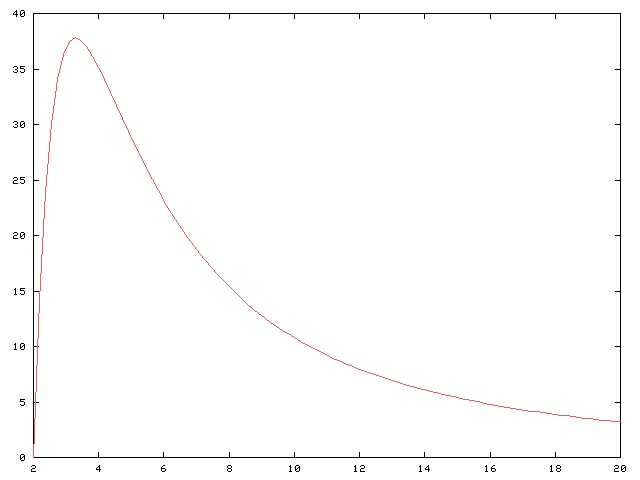As S increases, the probability falls off rapidly to zero, as you would expect, since the chance of rolling even one pair on a set of million-sided dice is quite slim.

The graph is quite typical, and each pattern has its own favorite kind of dice. Here's the corresponding graph and table for rolling the AABBCDEF pattern on eight dice:

 # ofsides Chance ofrolling AABBCDEF 6 9.00206 7 18.35970 8 25.23422 9 29.50469 10 31.75200 11 32.58759 12 32.49180 13 31.80697 14 30.76684 15 29.52744 16 28.19136 17 26.82506 18 25.47084 19 24.15487 20 22.89262 30 13.68370 40 8.85564 50 6.15085 100 1.80238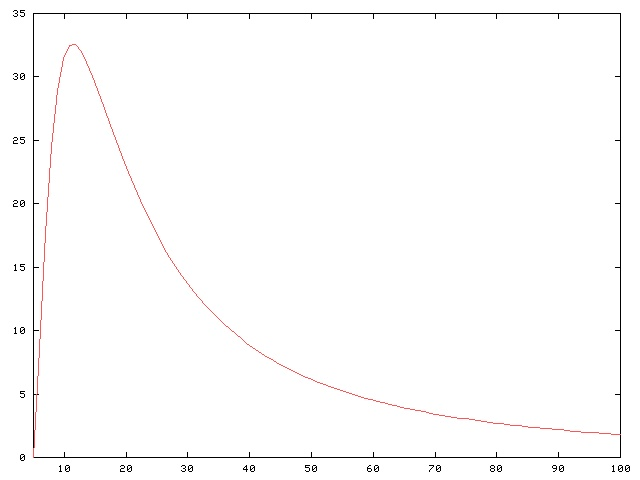As you can see, there is a sharp peak around N=11; you are more likely to roll two pair with eight 11-sided dice than you are with eight of any other sort of dice. Now if your boss catches you reading this article at work, you'll be prepared with an unassailable business justification for your behavior.

Returning to the discussion of poker hands, we might ask what the ranking of poker hands whould be, on the planet where a poker hand contains six cards instead of five. Does four of a kind beat three pair? Using the methods in this article, we can get a quick approximation. It will be something like this:

1. Two trips (AAABBB)
2. Overfull house (AAAABB)
3. Three pair
4. Four of a kind
5. Full house (AAABBC)
6. Three of a kind
7. Two pair
8. One pair
9. No pair
We'll need to calculate the values for straight and flush separately; they will be considerably rarer than in five-card poker.

I was going to end the article with tabulations of the number of different ways to roll each possible pattern, and the probabilities of getting them, but then I came to my senses. Instead of my running the program and pasting in the voluminous output, why not just let you run the program yourself, if you care to see the answers?

 Roll dice with sides each. Sort the results by frequency pattern.
Source code is here.